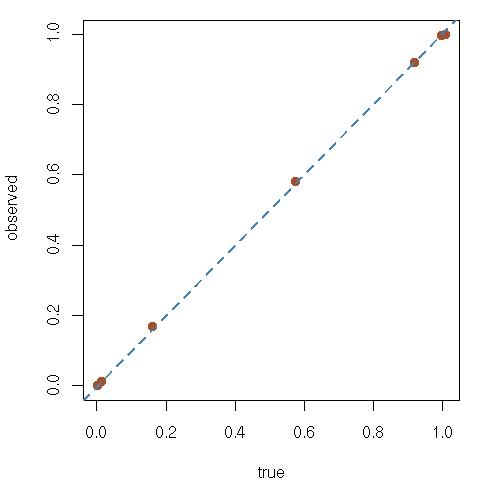# Random sudokus [test]

May 17, 2010
By

Want to share your content on R-bloggers? click here if you have a blog, or here if you don't.

Robin Ryder pointed out to me that 3 is indeed the absolute minimum one could observe because of the block constraint (bon sang, mais c’est bien sûr !). The distribution of the series of 3 digits being independent over blocks, the theoretical distribution under uniformity can easily be simulated:

#uniform distribution on the block diagonal
sheik=rep(0,9)
for (t in 1:10^6){
group=length(unique(c(sample(1:9,3),sample(1:9,3),sample(1:9,3))))
sheik[group]=sheik[group]+1
}

and it produces a result that is close enough to the one observed with the random sudoku generator. Actually, the exact distribution is available as (corrected on May 19!)

pdiag=c(1, #k=3
(3*6+3*6*4), #k=4
(3*choose(6,2)+3*6*5*choose(4,2)+3*choose(5,3)*choose(6,2)), #k=5
(choose(6,3)+3*6*choose(5,2)*4+3*choose(6,2)*choose(5,2)*4+
choose(6,3)*choose(6,3)),#k=6
(choose(3,2)*6*choose(5,3)+3*choose(6,2)*choose(4,2)*5+
choose(6,3)*choose(6,2)*3), #k=7
(3*choose(6,2)*4+choose(6,3)*6*choose(3,2)), #k=8
choose(6,3))/choose(9,3)^2 #k=9
choose(9,6))/choose(9,3)^2 #k=9

hence a better qq-plot:R-bloggers.com offers daily e-mail updates about R news and tutorials about learning R and many other topics. Click here if you're looking to post or find an R/data-science job.
Want to share your content on R-bloggers? click here if you have a blog, or here if you don't.

Tags: , , , , , , , ,